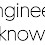# Heat transfer with internal Heat generation same temperature on both side graph plotting in python

In this case suppose we have a slab of and temperature on both side face of the is 60°C .Now there is some internal heat generation is going on inside the slab whose value is 80W /m^3 and the thickness of the slab is 1.2m . we will try to plot this on graph and see how to calculate temperature at different distance from one side of the slab

# i have made the code little shorter and better now it give more output
#CONSTRUCTIVE CRITICISM HIGHLY APPRECIATE

from matplotlib.pyplot import *

from numpy import *
from ipywidgets import interactive
t1 = t2 = 60 # temperature at both side of the slab is same unit in degree celcius
qg = 80 #w/m^3  internal heat generation
k = 1.148 # W/mK heat conduction coefficient
d = 1.2 # thickness of slab is 1.2 meter
def f(j):
x = linspace(0,1.2,360)
th = linspace(0,360,360)
t = qg/(2*k)*(d-j)*j +t1 # to get the value of temperature at certain distance
tth = qg/(2*k)*(d-x)*x+t1
axis([0,1.2,0,80]) #here 0 and 1.2 belong to x axis and 0 and 80 belong to y axis
a =[j]
b =[th]
title('Heat generation graph when temperature on both side is same')
xlabel('Distance in meter')
ylabel('temperature in degree celcius')
plot(x,tth,j,t,'o')

print('tempeature at x = ',j ,' is ',t,' C')

interactive_plot = interactive(f,j=(0,1.2,.01))
interactive_plot

1.Traceback (most recent call last):
File "C:/Users/A/AppData/Local/Programs/Python/Python310/r.py", line 22, in
print('tempeature at x = ',j ,' is ',t,' C')
NameError: name 'j' is not defined

1.